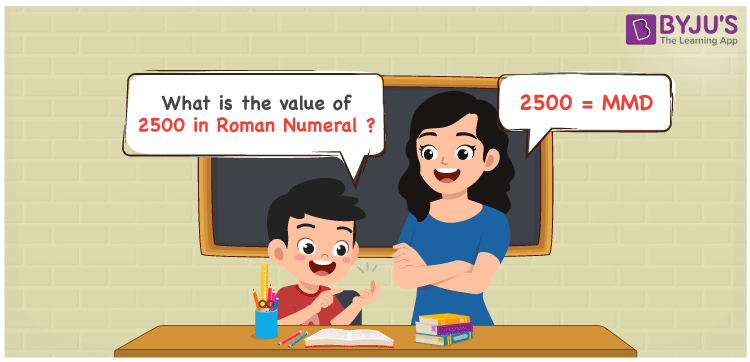# 2500 in Roman Numerals

2500 in Roman Numerals is MMD. Any Roman numeral uses only the 7 primary symbols which are I, V, X, L, C, D, and M, Where M represents 1000 and D represents 500. What are Roman numerals? can be referred for more details. The details on how to write 2500 are given in the next section of this article.

 Number Roman Numeral 2500 MMD

## How to Write 2500 in Roman Numerals?To convert 2500 in Roman Numerals, we need to represent 2500 as the sum of the fundamental symbols. 2500 can be broken down as the sum of 1000, 1000 and 500. Since M is the symbol for 1000, and D is the symbol for 500, it is expressed as MMD. Let us understand it numerically;

2500 = 1000 + 1000 + 500

2500 = M + M + D

2500 = MMD.

## Video Lesson on Roman Numerals## Frequently Asked Questions on 2500 in Roman Numerals

Q1

### How to write the number 2500 in Roman Numerals?

2500 in Roman Numerals is written as MMD.
Q2

### Do MMD and MMC represent Roman Numerals?

Yes. MMD represents Roman Numerals and it is the number 2500, whereas MMC is a Roman numeral which equals the number 2100.
Q3

### How to express the number Two Thousand Five Hundred in Roman numerals?

The number Two Thousand Five Hundred is expressed as MMD in Roman Numerals.